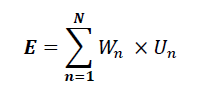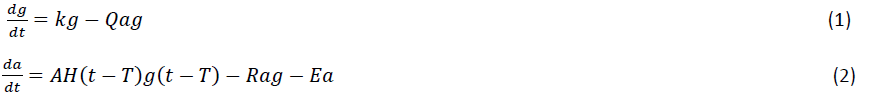# Using LabVIEW to Develop a Bioinspired Machine Learning Model for Security in a Wireless Sensor Network

"Fast algorithm engineering was one of the key benefits of using the NI platform. We developed and tested three algorithms in just one week."

- Heena Rathore, Indian Institute of Technology Jodhpur

The Challenge:
Implementing three existing algorithms to get a baseline for comparison and prove that a proposed wireless sensor network (WSN) algorithm is both cost-optimized and energy efficient.

The Solution:
Using NI LabVIEW system design software for rapid algorithm engineering of the swarm intelligence, artificial neural network, and weightings algorithms, and the newly proposed algorithm.

Author(s):
Heena Rathore - Indian Institute of Technology Jodhpur

Sensor nodes in a wireless sensor network (WSN) acquire data and send the data to the gateway wirelessly. The sensor nodes are remotely located so it is possible that someone could tamper with the physical signals connected to the sensors. Trust management systems for a WSN can detect this and assist with the decision-making process. Most of the algorithms for security in WSN applications are not cost-optimized or energy-efficient. We propose a new bio-inspired machine learning-based algorithm that overcomes both these issues. However, a key challenge in this research is getting a baseline for comparison from three existing algorithms. Many trust models are already developed including the weightings, artificial neural network, and swarm intelligence methods.

In the weightings method, initially every node is highly trusted and assigned a weight equal to one. The network is split between a group of sensor nodes and their forwarding nodes. Forwarding nodes collect all information provided by the sensor nodes and calculate an aggregation result using the weight assigned to each sensor node as given in the formula:Weights were updated for each of the sensor nodes based upon the variation of E with respect to U.

We implemented this algorithm in LabVIEW using a topology of nine sensor nodes, where initially each node was given a weight equal to one. Random data was generated between 10 and 20. Figure 1 shows the result depicting the rate of change of weights where the Y axis is the weights and the X axis is the time.

The artificial neural network approach in WSN involves calculating a trust value based on the present values as well as the past history of the neighboring nodes. The computing result is based on the interconnection pattern, the learning process, and the activation function, as shown in Figure 2. Based on the actual value received from the particular sensor node, the predicted value from estimation and prediction block, trust ratings are generated as shown in Figure 3. The Y axis is the measurement and the X axis is the time. The red line shows the predicted value of measurement and the black line shows the actual reading.

Swarm intelligence helps ants find the shortest path to a food source. Each ant deposits pheromones while traversing for the shortest path in such a way that the shorter the path, the more pheromone deposition. Ants converge on the shortest path as shown in Figure 4. Swarm intelligence is a trust model used to find the most reputable path leading to the most trustworthy node. We took 10 random active nodes in a grid in LabVIEW and computed the shortest path between the source and the destination, showing the nodes in between the path as the trustworthy nodes as shown in Figure 5. One is the source node and 10 is the destination node and the path shows the four, five, six, and nine nodes as the most trustworthy nodes. We used Dijsktra’s algorithm to implement the shortest path.

### Machine Learning Based and Human Immune System Model

We have divided our new proposed algorithm into two essential blocks—the machine learning module and the immune module. See the flowchart in Figure 6.

The machine learning module features three basic parts: the K-means, the support vector machine, and the anomaly detection engine. The K-means, an unsupervised machine learning method, works on the principle of finding a structure out of an unlabelled data set. The K-means algorithm groups data to make clusters as shown in Figure 7. For LabVIEW implementation, we used random data to generate two clusters. This was further fed to the support vector machine, which generally takes labeled data and creates the decision boundary by extrapolating the data field. Figure 8 shows red data points as the malicious data points and green data points as the benevolent data points. We used the machine learning module in LabVIEW for implementation purposes. The anomaly detection engine determined the boundary values between the two regions. The mean and standard deviation of the benevolent region was calculated to get the Gaussian probability distribution of data points as shown in Figure 9. We maintained the threshold so that the value below that was considered malicious. The outcome of the machine learning module was the set of malicious data points.

In 1977, Dibrov and others devised a model to study the rate of change of antibodies and antigen. The Dibrov Model consists of coupled equations for the antibody quantity (a) and the antigen quantity (g). Consider the set of equations describing antigen-antibody interactions:Equation 1 and 2 are the rate of change of the antigen and antibody, respectively. Also K, Q, A, R, and E are rate constants. K is the overall growth rate of the antigen. H(t) in equation 2 is the Heaviside step function in which the value is zero for a negative argument and one for a positive argument. When we carried out simulations in LabVIEW using the Runge-Kutta (variable) method for solving the differential equations, we saw results as shown in Figure 10. It shows the graph of the rate of change of antigen and antibody as a function of time, for values of K=0.01, Q=1, A=1, R=1, and E=1 with initial conditions a0=0 and g0=1. This shows that the antigen count linearly increases and when the body comes to know about it, the B cells start producing antibodies. When the antigen antibody complex forms, the count of antigen decreases linearly and the rate of change of antibody becomes constant.

In the immune module, we based the virtual antibody production analogous to antibody production by B cells in the human immune system on the Dibrov Model. The module focuses on giving correct readings even if the node has become malicious. For this, we assign weights to the measurement values and these weights are proportional to the antigen values determined from solving the differential equations. Prior to the malicious node detection, weights are assigned as one. After the malicious node is detected, the expected measurement value depends on the previous measurements and the weights decrease proportional to the antigen values. After the node becomes malicious, the proposed algorithm slowly decreases or increases the sampling interval to zero, thus preserving the stability of the system while removing the impact of the malicious node. We implemented this new algorithm using the NI WSN-9791 Ethernet gateway and NI WSN-3212 thermocouple input node.

### Benefits of Using NI Products

Fast algorithm engineering was one of the key benefits of using the NI platform. We developed and tested three algorithms in just one week. This gave us a quick baseline for comparing our newly proposed algorithm. Implementing the new algorithm in software and then testing it on real-world signals through hardware was also simple with the NI platform.

Author Information:
Heena Rathore
Indian Institute of Technology Jodhpur
29, Bhairav Dham Colony, Sector-3, Hiran Magri
Udaipur
India
Tel: 9413695821
heena7sept@iitj.ac.in

## Explore the NI Developer CommunityDiscover and collaborate on the latest example code and tutorials with a worldwide community of engineers and scientists.

## Who is National Instruments?

National Instruments provides a graphical system design platform for test, control, and embedded design applications that is transforming the way engineers and scientists design, prototype, and deploy systems.